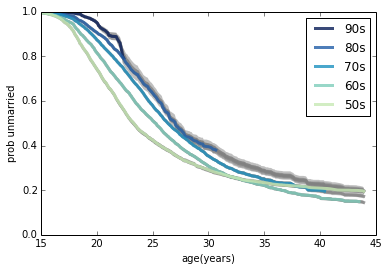This notebook contains examples related to survival analysis, based on Chapter 13 of
Think Stats, 2nd Edition
by Allen Downey
available from thinkstats2.com

In :
from __future__ import print_function, division

import nsfg
import survival

import thinkstats2
import thinkplot

import pandas
import numpy
from lifelines import KaplanMeierFitter
from collections import defaultdict

import matplotlib.pyplot as pyplot

%matplotlib inline


The first example looks at pregnancy lengths for respondents in the National Survey of Family Growth (NSFG). This is the easy case, because we can directly compute the CDF of pregnancy length; from that we can get the survival function:

In :
preg = nsfg.ReadFemPreg()
complete = preg.query('outcome in [1, 3, 4]').prglngth

In :
cdf = thinkstats2.Cdf(complete, label='cdf')
sf = survival.SurvivalFunction(cdf, label='survival')
thinkplot.Plot(sf)
thinkplot.Config(xlabel='duration (weeks)', ylabel='survival function')
#thinkplot.Save(root='survival_talk1', formats=['png'])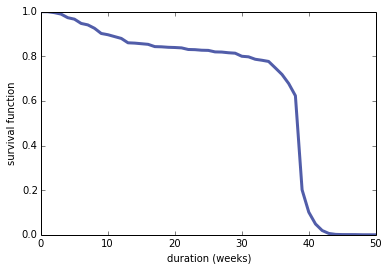About 17% of pregnancies end in the first trimester, but a large majorty pregnancies that exceed 13 weeks go to full term.

Next we can use the survival function to compute the hazard function.

In :
hf = sf.MakeHazard(label='hazard')
thinkplot.Plot(hf)
thinkplot.Config(xlabel='duration (weeks)', ylabel='hazard function', ylim=[0, 0.75], loc='upper left')
#thinkplot.Save(root='survival_talk2', formats=['png'])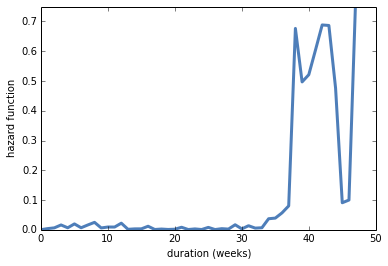The hazard function shows the same pattern: the lowest hazard in the second semester, and by far the highest hazard around 30 weeks.

We can also use the survival curve to compute mean remaining lifetime as a function of how long the pregnancy has gone.

In :
rem_life = sf.RemainingLifetime()
thinkplot.Plot(rem_life)
thinkplot.Config(xlabel='weeks', ylabel='mean remaining weeks', legend=False)
#thinkplot.Save(root='survival_talk3', formats=['png'])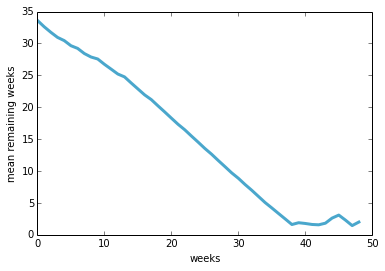For 38 weeks, the finish line approaches nearly linearly. But at 39 weeks, the expected remaining time levels off abruptly. After that, each week that passes brings the finish line no closer.

I started with pregnancy lengths because they represent the easy case where the distribution of lifetimes is known. But often in observational studies we have a combination of complete cases, where the lifetime is known, and ongoing cases where we have a lower bound on the lifetime.

As an example, we'll look at the time until first marriage for women in the NSFG.

In :
resp = survival.ReadFemResp2002()
len(resp)

Out:
7643

For complete cases, we know the respondent's age at first marriage. For ongoing cases, we have the respondent's age when interviewed.

In :
complete = resp[resp.evrmarry == 1].agemarry
ongoing = resp[resp.evrmarry == 0].age


There are only a few cases with unknown marriage dates.

In :
nan = complete[numpy.isnan(complete)]
len(nan)

Out:
37

EstimateHazardFunction is an implementation of Kaplan-Meier estimation.

With an estimated hazard function, we can compute a survival function.

In :
hf = survival.EstimateHazardFunction(complete, ongoing)
sf = hf.MakeSurvival()


Here's the hazard function:

In :
thinkplot.Plot(hf)
thinkplot.Config(xlabel='age (years)', ylabel='hazard function', legend=False)
#thinkplot.Save(root='survival_talk4', formats=['png'])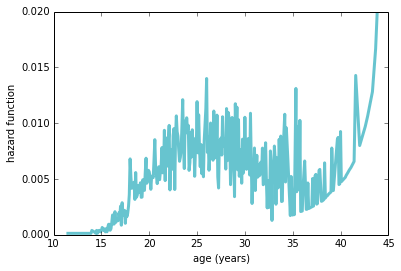As expected, the hazard function is highest in the mid-20s. The function increases again after 35, but that is an artifact of the estimation process and a misleading visualization. Making a better representation of the hazard function is on my TODO list.

Here's the survival function:

In :
thinkplot.Plot(sf)
thinkplot.Config(xlabel='age (years)',
ylabel='prob unmarried',
ylim=[0, 1],
legend=False)
#thinkplot.Save(root='survival_talk5', formats=['png'])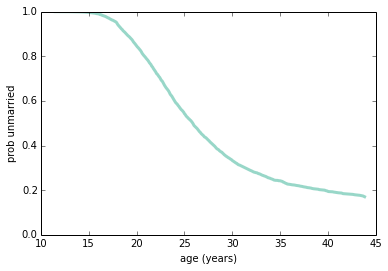The survival function naturally smooths out the noisiness in the hazard function.

With the survival curve, we can also compute the probability of getting married before age 44, as a function of current age.

In :
ss = sf.ss
end_ss = ss[-1]
prob_marry44 = (ss - end_ss) / ss
thinkplot.Plot(sf.ts, prob_marry44)
thinkplot.Config(xlabel='age (years)', ylabel='prob marry before 44', ylim=[0, 1], legend=False)
#thinkplot.Save(root='survival_talk6', formats=['png'])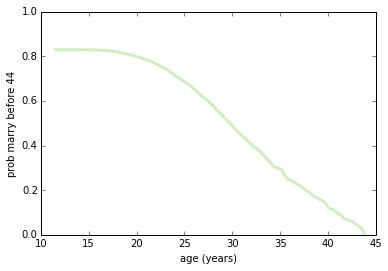After age 20, the probability of getting married drops off nearly linearly.

We can also compute the median time until first marriage as a function of age.

In :
func = lambda pmf: pmf.Percentile(50)
thinkplot.Plot(rem_life)
thinkplot.Config(ylim=[0, 15],
xlim=[11, 31],
xlabel='age (years)',
ylabel='median remaining years')
#thinkplot.Save(root='survival_talk7', formats=['png'])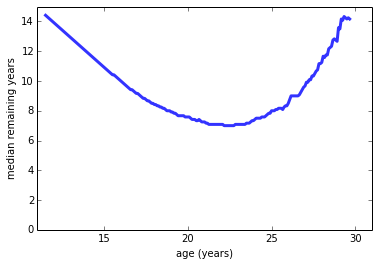At age 11, young women are a median of 14 years away from their first marriage. At age 23, the median has fallen to 7 years. But an never-married woman at 30 is back to a median remaining time of 14 years.

I also want to demonstrate lifelines, which is a Python module that provides Kaplan-Meier estimation and other tools related to survival analysis.

To use lifelines, we have to get the data into a different format. First I'll add a column to the respondent DataFrame with "event times", meaning either age at first marriage OR age at time of interview.

In :
resp['event_times'] = resp.age
resp['event_times'][resp.evrmarry == 1] = resp.agemarry
len(resp)

Out:
7643

Lifelines doesn't like NaNs, so let's get rid of them:

In :
cleaned = resp.dropna(subset=['event_times'])
len(cleaned)

Out:
7606

Now we can use the KaplanMeierFitter, passing the series of event times and a series of booleans indicating which events are complete and which are ongoing:

In :
kmf = KaplanMeierFitter()
kmf.fit(cleaned.event_times, cleaned.evrmarry)

Out:
<lifelines.KaplanMeierFitter: fitted with 7606 observations, 3517 censored>

Here are the results from my implementation compared with the results from Lifelines.

In :
thinkplot.Plot(sf)
thinkplot.Config(xlim=[0, 45], legend=False)
pyplot.grid()
kmf.survival_function_.plot()

Out:
<matplotlib.axes.AxesSubplot at 0x7f7c2955ba90>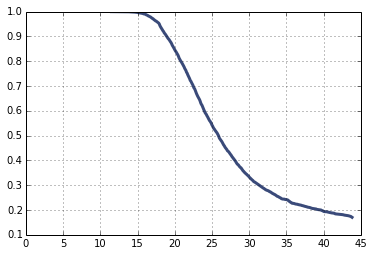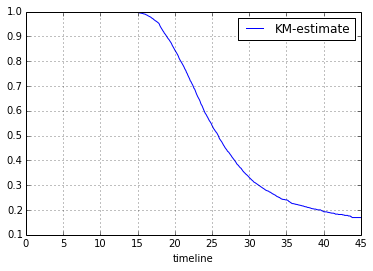They are at least visually similar. Just to double check, I ran a small example:

In :
complete = [1, 2, 3]
ongoing = [2.5, 3.5]


Here's the hazard function:

In :
hf = survival.EstimateHazardFunction(complete, ongoing)
hf.series

Out:
1    0.20
2    0.25
3    0.50
dtype: float64

And the survival function.

In :
sf = hf.MakeSurvival()
sf.ts, sf.ss

Out:
(array([1, 2, 3]), array([ 0.8,  0.6,  0.3]))

My implementation only evaluate the survival function at times when a completed event occurred.

Next I'll reformat the data for lifelines:

In :
T = pandas.Series(complete + ongoing)
E = [1, 1, 1, 0, 0]


And run the KaplanMeier Fitter:

In :
kmf = KaplanMeierFitter()
kmf.fit(T, E)
kmf.survival_function_

Out:
KM-estimate
timeline
0.0 1.0
1.0 0.8
2.0 0.6
2.5 0.6
3.0 0.3
3.5 0.3

The results are the same, except that the Lifelines implementation evaluates the survival function at all event times, complete or not.

Next, I'll use additional data from the NSFG to investigate "marriage curves" for successive generations of women.

Here's data from the last 4 cycles of the NSFG:

In :
resp5 = survival.ReadFemResp1995()


This function takes a respondent DataFrame and estimates survival curves:

In :
def EstimateSurvival(resp):
"""Estimates the survival curve.

resp: DataFrame of respondents

returns: pair of HazardFunction, SurvivalFunction
"""
complete = resp[resp.evrmarry == 1].agemarry
ongoing = resp[resp.evrmarry == 0].age

hf = survival.EstimateHazardFunction(complete, ongoing)
sf = hf.MakeSurvival()

return hf, sf


This function takes a list of respondent files, resamples them, groups by decade, optionally generates predictions, and returns a map from group name to a list of survival functions (each based on a different resampling):

In :
def ResampleSurvivalByDecade(resps, iters=101, predict_flag=False, omit=[]):
"""Makes survival curves for resampled data.

resps: list of DataFrames
iters: number of resamples to plot
predict_flag: whether to also plot predictions

returns: map from group name to list of survival functions
"""
sf_map = defaultdict(list)

# iters is the number of resampling runs to make
for i in range(iters):

# we have to resample the data from each cycles separately
samples = [thinkstats2.ResampleRowsWeighted(resp)
for resp in resps]

# then join the cycles into one big sample
sample = pandas.concat(samples, ignore_index=True)

# and estimate (hf, sf) for each group
hf_map = grouped.apply(lambda group: EstimateSurvival(group))

if predict_flag:

# extract the sf from each pair and acculumulate the results
for name, (hf, sf) in hf_map.iteritems():
sf_map[name].append(sf)

return sf_map


And here's how the predictions work:

In :
def MakePredictionsByDecade(hf_map, **options):
"""Extends a set of hazard functions and recomputes survival functions.

For each group in hf_map, we extend hf and recompute sf.

hf_map: map from group name to (HazardFunction, SurvivalFunction)
"""
# TODO: this only works if the names and values are in increasing order,
# which is true when hf_map is a GroupBy object, but not generally
# true for maps.
names = hf_map.index.values
hfs = [hf for (hf, sf) in hf_map.values]

# extend each hazard function using data from the previous cohort,
# and update the survival function
for i, hf in enumerate(hfs):
if i > 0:
hf.Extend(hfs[i-1])
sf = hf.MakeSurvival()
hf_map[names[i]] = hf, sf


This function takes a list of survival functions and returns a confidence interval:

In :
def MakeSurvivalCI(sf_seq, percents):

# find the union of all ts where the sfs are evaluated
ts = set()
for sf in sf_seq:
ts |= set(sf.ts)

ts = list(ts)
ts.sort()

# evaluate each sf at all times
ss_seq = [sf.Probs(ts) for sf in sf_seq]

# return the requested percentiles from each column
rows = thinkstats2.PercentileRows(ss_seq, percents)
return ts, rows


Make survival curves without predictions:

In :
resps = [resp5, resp6, resp7, resp8]


Make survival curves with predictions:

In :
resps = [resp5, resp6, resp7, resp8]


This function plots survival curves:

In :
def PlotSurvivalFunctionByDecade(sf_map, predict_flag=False):
thinkplot.PrePlot(len(sf_map))

for name, sf_seq in sorted(sf_map.iteritems(), reverse=True):
ts, rows = MakeSurvivalCI(sf_seq, [10, 50, 90])
thinkplot.FillBetween(ts, rows, rows, color='gray')
if predict_flag:
thinkplot.Plot(ts, rows, color='gray')
else:
thinkplot.Plot(ts, rows, label='%d0s'%name)

thinkplot.Config(xlabel='age(years)', ylabel='prob unmarried',
xlim=[15, 45], ylim=[0, 1], legend=True, loc='upper right')


Now we can plot results without predictions:

In :
PlotSurvivalFunctionByDecade(sf_map)
#thinkplot.Save(root='survival_talk8', formats=['png'])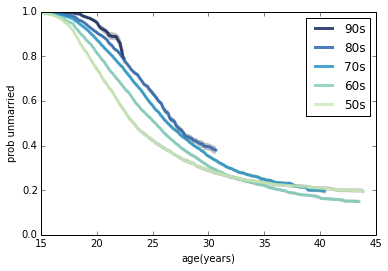And plot again with predictions:

In :
PlotSurvivalFunctionByDecade(sf_map_pred, predict_flag=True)